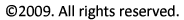Problem Statement:

A small propeller-driven airplane is flying at a speed where its total drag is 1250 N. The aircraft engine is burning 0.44 L/min (4.4 x 10-4 m3/min) of fuel. The fuel has a density of 700 kg/m3 and an energy density of 43.5 MJ/kg. The thermal efficiency of the engine is ηth = 25% and the propulsive efficiency of the propeller is ηp = 80%. How fast is the airplane flying?

Solution:

From the definition of overall efficiency we have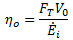But for steady flight, FT = FD. Also, we know that ηo = ηthηp. Substituting all of this into the above equation and solving for the airplane speed gives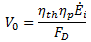All we need to know is how to find the energy input. The energy input comes from the chemical energy in the fuel and is computed as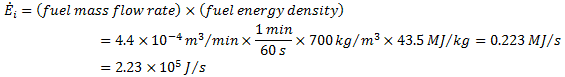Thus, we compute the airplane speed as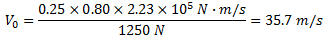or, about 80 mph. Note that we have used the fact that 1 J = 1 N m/s in the calculation.